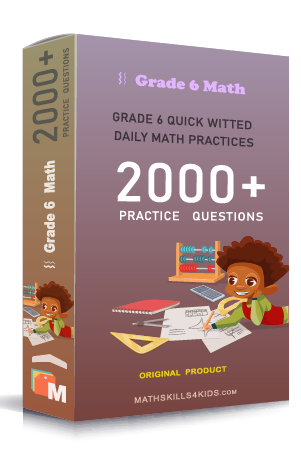Subject
Math

Resource Type
Worksheets, Printables, Homeschool

Format
PDF (15.1 MB | 18 pages → Exercises + Solutions)

• ### -15% OFF Over \$100

Promo Code: TOPLA

After Purchase

\$2.15

This package allows you to practice the following skills

Adding and subtracting fractions with the same denominator

Adding and subtracting fractions with the same denominator word problems

Adding and subtracting fractions with unlike denominator

Adding and subtracting fractions with unlike denominator word problems

Inequalities with addition and subtract of like and unlike fraction

Adding and subtracting mixed numbers word problems

Comparing fractions with like and unlike denominator

Estimating sums and differences of mixed numbers

Solving maps with fractional distances

This product is a part of the Mega Pack
* Grade 6 quick-witted daily math practices *